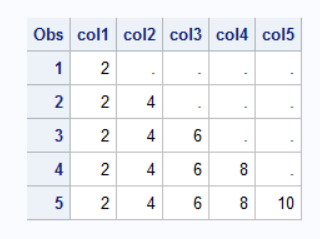## Nested Loop in SAS: Issue with index values

Explain why I am getting all the EVEN values when I am doing i+j as a final result in the nested loop:

``````data floyd;
array arr(*) col1-col5;
do i=1 to 5;
do j=1 to i;
arr(i) = i+j;
end;
output;
end;
drop i j;
run;
proc print; run;``````As per my understanding output has to be :

Expected output:and so on.........

1 ACCEPTED SOLUTION

Accepted Solutions

## Re: Nested Loop in SAS: Issue with index values

No.

You seem to have confused which index variable represents the observation number (aka ROW) and which one represents the variable number (aka COLUMN).

It would also be clearer to empty the array after you write it to see what you are really doing.

``````data floyd;
array arr[*] col1-col5;
do i=1 to 5;
do j=1 to i;
arr[i] = i+j;
end;
output;
call missing(of arr[*]);
end;
drop i j;
run;``````

Result

```Obs    col1    col2    col3    col4    col5

1       2       .       .       .       .
2       .       4       .       .       .
3       .       .       6       .       .
4       .       .       .       8       .
5       .       .       .       .      10
```

So when I=2 you write two different values into ARR, so only the final one, 4, remains.

It becomes clearer is you use ROW and COL as the index variable names.

Now it become clearer that you wanted:

``arr[col] = row+col;``

``arr[row] = row+col;``

Try

``````data floyd;
array arr[*] col1-col5;
do row=1 to 5;
do col=1 to row;
arr[col] = row+col;
end;
output;
end;
drop row col;
run;
proc print; run;``````
2 REPLIES 2

## Re: Nested Loop in SAS: Issue with index values

No.

You seem to have confused which index variable represents the observation number (aka ROW) and which one represents the variable number (aka COLUMN).

It would also be clearer to empty the array after you write it to see what you are really doing.

``````data floyd;
array arr[*] col1-col5;
do i=1 to 5;
do j=1 to i;
arr[i] = i+j;
end;
output;
call missing(of arr[*]);
end;
drop i j;
run;``````

Result

```Obs    col1    col2    col3    col4    col5

1       2       .       .       .       .
2       .       4       .       .       .
3       .       .       6       .       .
4       .       .       .       8       .
5       .       .       .       .      10
```

So when I=2 you write two different values into ARR, so only the final one, 4, remains.

It becomes clearer is you use ROW and COL as the index variable names.

Now it become clearer that you wanted:

``arr[col] = row+col;``

``arr[row] = row+col;``

Try

``````data floyd;
array arr[*] col1-col5;
do row=1 to 5;
do col=1 to row;
arr[col] = row+col;
end;
output;
end;
drop row col;
run;
proc print; run;``````

## Re: Nested Loop in SAS: Issue with index values

Very depth explanation, Thank you.

Discussion stats
• 2 replies
• 131 views
• 1 like
• 2 in conversation